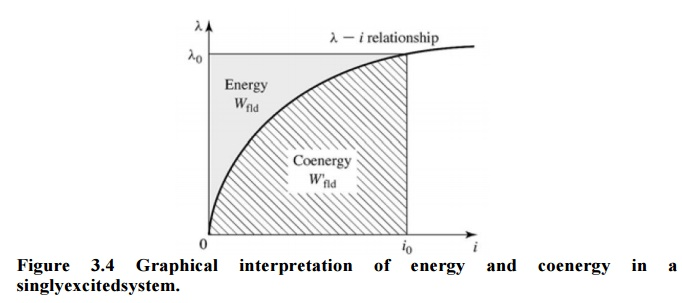Home | | Electrical Machines I | The Co Energy

# The Co Energy

Coenergy: Here the force can be obtained directly as a function of the current. The selection of energy or coenergy as the state function is purely a matter of convenience.

The  Co Energy

The magnetic stored energy is a state function, determined uniquely by the values of the independent state variables λ and x

Coenergy: Here the force can be obtained directly as a function of the current. The selection of energy or coenergy as the state function is purely a matter of convenience.

For a magnetically-linear system, the energy and coenergy (densities) are numerically equal:

Wfld+W’fld=λiThe force acts in a direction to decrease the magnetic field stored energy at constant flux or to increase the coenergy at constant current. In a singly-excited device, the force acts to increase the inductance by pulling on members so as to reduce the reluctance of the magnetic path linking the winding.

Study Material, Lecturing Notes, Assignment, Reference, Wiki description explanation, brief detail
Electrical machines : Electromechanical Energy Conversion and Concepts in Rotating Machines : The Co Energy |